Next: Solutions of the Klein-Gordon Up: Klein-Gordon Equation Previous: Wave Equation for a

# Lorentz Invariance

We now study the Lorentz invariance of the Klein-Gordon equation. The operator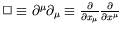is invariant under a Lorentz transformation because it is a scalar product of 4-vectors,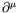. Also, the mass,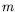, is a scalar. Now consider a transformation from an unprimed system to a primed system. In the transformed primed system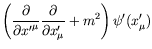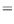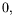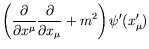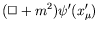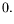(4.12)

Since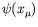and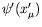refer to the same space-time point and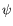is a scalar, the Klein-Gordon equation is Lorentz invariant. Notice thatand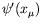are different and are related by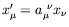. Alsoandrefer to two different points with coordinates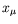in the old and new system, respectively.

Since the Klein-Gordon operator does not change under continuous Lorentz transformations, we can reason that the wave function is multiplied by a factor with absolute value of unity in these transformations. In the case of the coordinate transformation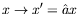(where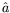is the Lorentz operator) the transformation law of the wave function is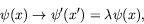(4.13)

with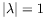. Sinceis real,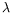must be real and hence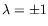.

If the Lorentz transformation is continuous (ie. rotation in 4-space),depends continuously on some variables, say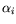. For all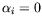, we must have the identity transformation and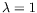holds. A wave function which does not change under spatial rotations can describe a particle with spin-0.

For space inversion, which is a discrete Lorentz transformation,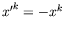and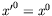. Applying the space inversion operator twice leads to the identity transformation. Therefore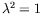or. We define two states:

for the case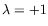,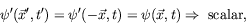(4.14)

for the case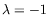,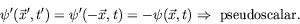(4.15)

Therefore solutions of the Klein-Gordon equation are scalar or pseudoscalar, ie. invariant under spatial rotations and proper Lorentz transformations, and are invariant (scalar) or change sign (pseudoscalar) under space inversion. The pi-meson (pion) is an example of a pseudoscalar meson that obeys the Klein-Gordon equation.Next: Solutions of the Klein-Gordon Up: Klein-Gordon Equation Previous: Wave Equation for a
Douglas M. Gingrich (gingrich@ ualberta.ca)
2004-03-18